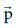Home | | Physics 11th std | Kinetic energy

# Kinetic energy

Kinetic energy is the energy possessed by a body by virtue of its motion.

Kinetic energy

Kinetic energy is the energy possessed by a body by virtue of its motion. All moving objects have kinetic energy. A body that is in motion has the ability to do work. For example a hammer kept at rest on a nail does not push the nail into the wood. Whereas the same hammer when it strikes the nail, draws the nail into the wood as shown in Figure 4.7. Kinetic energy is measured by the amount of work that the body can perform before it comes to rest. The amount of work done by a moving body depends both on the mass of the body and the magnitude of its velocity. A body which is not in motion does not have kinetic energy.

## Work-Kinetic Energy Theorem

Work and energy are equivalents. This is true in the case of kinetic energy also. To prove this, let us consider a body of mass m at rest on a frictionless horizontal surface.

The work (W) done by the constant force (F) for a displacement (s) in the same direction is,The constant force is given by the equation,The third equation of motion (refer section 2.10.3) can be written as,The expression for kinetic energy:

The term ½ mv2 in the above equation is the kinetic energy of the body of mass (m) moving with velocity (v).Kinetic energy of the body is always positive. From equations (4.10) and (4.11)The expression on the right hand side (RHS) of equation (4.12) is the change in kinetic energy (∆KE) of the body.This implies that the work done by the force on the body changes the kinetic energy of the body. This is called work-kinetic energy theorem.

The work-kinetic energy theorem implies the following.

i.               If the work done by the force on the body is positive then its kinetic energy increases.

ii.               If the work done by the force on the body is negative then its kinetic energy decreases.

iii.               If there is no work done by the force on the body then there is no change in its kinetic energy, which means that the body has moved at constant speed provided its mass remains constant.

## Relation between Momentum and Kinetic Energy

Consider an object of mass m moving with a velocity. Then its linear momentum isand its kinetic energy, KE =  ½ mv2 .Multiplying both the numerator and denominator of equation (4.13) by mass, mwhereis the magnitude of the momentum. The magnitude of the linear momentum can be obtained byNote that if kinetic energy and mass are given, only the magnitude of the momentum can be calculated but not the direction of momentum. It is because the kinetic energy and mass are scalars.

## Solved Example Problems for  Kinetic energy

### Example 4.7

Two objects of masses 2 kg and 4 kg are moving with the same momentum of 20 kg m s-1.

a. Will they have same kinetic energy?

b. Will they have same speed?

### Solution

a. The kinetic energy of the mass is given byNote that KE1 ≠ KE2 i.e., even though both are having the same momentum, the kinetic energy of both masses is not the same. The kinetic energy of the heavier object has lesser kinetic energy than smaller mass. It is because the kinetic energy is inversely proportional to the mass (KE  1/m) for a given momentum.

b. As the momentum, p = mv, the two objects will not have same speed.

Study Material, Lecturing Notes, Assignment, Reference, Wiki description explanation, brief detail
11th Physics : UNIT 4 : Work, Energy and Power : Kinetic energy |